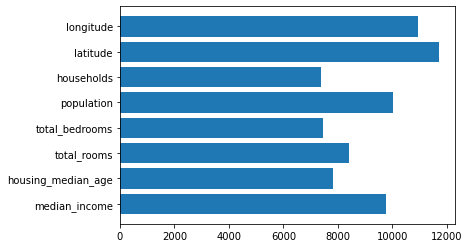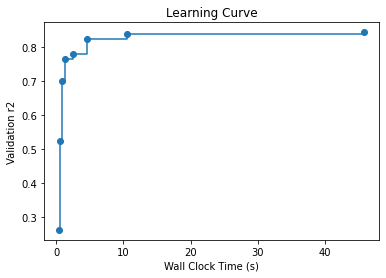# AutoML for LightGBM

### Prerequisites for this example​

Install the [notebook] option.

pip install "flaml[notebook]"

This option is not necessary in general.

### Use built-in LGBMEstimator​

from flaml import AutoMLfrom flaml.automl.data import load_openml_dataset# Download [houses dataset](https://www.openml.org/d/537) from OpenML. The task is to predict median price of the house in the region based on demographic composition and a state of housing market in the region.X_train, X_test, y_train, y_test = load_openml_dataset(dataset_id=537, data_dir='./')automl = AutoML()settings = {    "time_budget": 60,  # total running time in seconds    "metric": 'r2',  # primary metrics for regression can be chosen from: ['mae','mse','r2']    "estimator_list": ['lgbm'],  # list of ML learners; we tune lightgbm in this example    "task": 'regression',  # task type    "log_file_name": 'houses_experiment.log',  # flaml log file    "seed": 7654321,  # random seed}automl.fit(X_train=X_train, y_train=y_train, **settings)

#### Sample output​

[flaml.automl: 11-15 19:46:44] {1485} INFO - Data split method: uniform[flaml.automl: 11-15 19:46:44] {1489} INFO - Evaluation method: cv[flaml.automl: 11-15 19:46:44] {1540} INFO - Minimizing error metric: 1-r2[flaml.automl: 11-15 19:46:44] {1577} INFO - List of ML learners in AutoML Run: ['lgbm'][flaml.automl: 11-15 19:46:44] {1826} INFO - iteration 0, current learner lgbm[flaml.automl: 11-15 19:46:44] {1944} INFO - Estimated sufficient time budget=3232s. Estimated necessary time budget=3s.[flaml.automl: 11-15 19:46:44] {2029} INFO -  at 0.5s,  estimator lgbm's best error=0.7383, best estimator lgbm's best error=0.7383[flaml.automl: 11-15 19:46:44] {1826} INFO - iteration 1, current learner lgbm[flaml.automl: 11-15 19:46:44] {2029} INFO -  at 0.6s,  estimator lgbm's best error=0.4774, best estimator lgbm's best error=0.4774[flaml.automl: 11-15 19:46:44] {1826} INFO - iteration 2, current learner lgbm[flaml.automl: 11-15 19:46:44] {2029} INFO -  at 0.7s,  estimator lgbm's best error=0.4774, best estimator lgbm's best error=0.4774[flaml.automl: 11-15 19:46:44] {1826} INFO - iteration 3, current learner lgbm[flaml.automl: 11-15 19:46:44] {2029} INFO -  at 0.9s,  estimator lgbm's best error=0.2985, best estimator lgbm's best error=0.2985[flaml.automl: 11-15 19:46:44] {1826} INFO - iteration 4, current learner lgbm[flaml.automl: 11-15 19:46:45] {2029} INFO -  at 1.3s,  estimator lgbm's best error=0.2337, best estimator lgbm's best error=0.2337[flaml.automl: 11-15 19:46:45] {1826} INFO - iteration 5, current learner lgbm[flaml.automl: 11-15 19:46:45] {2029} INFO -  at 1.4s,  estimator lgbm's best error=0.2337, best estimator lgbm's best error=0.2337[flaml.automl: 11-15 19:46:45] {1826} INFO - iteration 6, current learner lgbm[flaml.automl: 11-15 19:46:46] {2029} INFO -  at 2.5s,  estimator lgbm's best error=0.2219, best estimator lgbm's best error=0.2219[flaml.automl: 11-15 19:46:46] {1826} INFO - iteration 7, current learner lgbm[flaml.automl: 11-15 19:46:46] {2029} INFO -  at 2.9s,  estimator lgbm's best error=0.2219, best estimator lgbm's best error=0.2219[flaml.automl: 11-15 19:46:46] {1826} INFO - iteration 8, current learner lgbm[flaml.automl: 11-15 19:46:48] {2029} INFO -  at 4.5s,  estimator lgbm's best error=0.1764, best estimator lgbm's best error=0.1764[flaml.automl: 11-15 19:46:48] {1826} INFO - iteration 9, current learner lgbm[flaml.automl: 11-15 19:46:54] {2029} INFO -  at 10.5s, estimator lgbm's best error=0.1630, best estimator lgbm's best error=0.1630[flaml.automl: 11-15 19:46:54] {1826} INFO - iteration 10, current learner lgbm[flaml.automl: 11-15 19:46:56] {2029} INFO -  at 12.4s, estimator lgbm's best error=0.1630, best estimator lgbm's best error=0.1630[flaml.automl: 11-15 19:46:56] {1826} INFO - iteration 11, current learner lgbm[flaml.automl: 11-15 19:47:13] {2029} INFO -  at 29.0s, estimator lgbm's best error=0.1630, best estimator lgbm's best error=0.1630[flaml.automl: 11-15 19:47:13] {1826} INFO - iteration 12, current learner lgbm[flaml.automl: 11-15 19:47:15] {2029} INFO -  at 31.1s, estimator lgbm's best error=0.1630, best estimator lgbm's best error=0.1630[flaml.automl: 11-15 19:47:15] {1826} INFO - iteration 13, current learner lgbm[flaml.automl: 11-15 19:47:29] {2029} INFO -  at 45.8s, estimator lgbm's best error=0.1564, best estimator lgbm's best error=0.1564[flaml.automl: 11-15 19:47:33] {2242} INFO - retrain lgbm for 3.2s[flaml.automl: 11-15 19:47:33] {2247} INFO - retrained model: LGBMRegressor(colsample_bytree=0.8025848209352517,              learning_rate=0.09100963138990374, max_bin=255,              min_child_samples=42, n_estimators=363, num_leaves=216,              reg_alpha=0.001113000336715291, reg_lambda=76.50614276906414,              verbose=-1)[flaml.automl: 11-15 19:47:33] {1608} INFO - fit succeeded[flaml.automl: 11-15 19:47:33] {1610} INFO - Time taken to find the best model: 45.75616669654846[flaml.automl: 11-15 19:47:33] {1624} WARNING - Time taken to find the best model is 76% of the provided time budget and not all estimators' hyperparameter search converged. Consider increasing the time budget.

#### Retrieve best config​

print('Best hyperparmeter config:', automl.best_config)print('Best r2 on validation data: {0:.4g}'.format(1-automl.best_loss))print('Training duration of best run: {0:.4g} s'.format(automl.best_config_train_time))print(automl.model.estimator)# Best hyperparmeter config: {'n_estimators': 363, 'num_leaves': 216, 'min_child_samples': 42, 'learning_rate': 0.09100963138990374, 'log_max_bin': 8, 'colsample_bytree': 0.8025848209352517, 'reg_alpha': 0.001113000336715291, 'reg_lambda': 76.50614276906414}# Best r2 on validation data: 0.8436# Training duration of best run: 3.229 s# LGBMRegressor(colsample_bytree=0.8025848209352517,#               learning_rate=0.09100963138990374, max_bin=255,#               min_child_samples=42, n_estimators=363, num_leaves=216,#               reg_alpha=0.001113000336715291, reg_lambda=76.50614276906414,#               verbose=-1)

#### Plot feature importance​

import matplotlib.pyplot as pltplt.barh(automl.feature_names_in_, automl.feature_importances_)#### Compute predictions of testing dataset​

y_pred = automl.predict(X_test)print('Predicted labels', y_pred)# Predicted labels [143391.65036562 245535.13731811 153171.44071629 ... 184354.52735963#  235510.49470445 282617.22858956]

#### Compute different metric values on testing dataset​

from flaml.automl.ml import sklearn_metric_loss_scoreprint('r2', '=', 1 - sklearn_metric_loss_score('r2', y_pred, y_test))print('mse', '=', sklearn_metric_loss_score('mse', y_pred, y_test))print('mae', '=', sklearn_metric_loss_score('mae', y_pred, y_test))# r2 = 0.8505434326526395# mse = 1975592613.138005# mae = 29471.536046068788

#### Compare with untuned LightGBM​

from lightgbm import LGBMRegressorlgbm = LGBMRegressor()lgbm.fit(X_train, y_train)y_pred = lgbm.predict(X_test)from flaml.automl.ml import sklearn_metric_loss_scoreprint('default lgbm r2', '=', 1 - sklearn_metric_loss_score('r2', y_pred, y_test))# default lgbm r2 = 0.8296179648694404

#### Plot learning curve​

How does the model accuracy improve as we search for different hyperparameter configurations?

from flaml.automl.data import get_output_from_logimport numpy as nptime_history, best_valid_loss_history, valid_loss_history, config_history, metric_history =    get_output_from_log(filename=settings['log_file_name'], time_budget=60)plt.title('Learning Curve')plt.xlabel('Wall Clock Time (s)')plt.ylabel('Validation r2')plt.step(time_history, 1 - np.array(best_valid_loss_history), where='post')plt.show()### Use a customized LightGBM learner​

The native API of LightGBM allows one to specify a custom objective function in the model constructor. You can easily enable it by adding a customized LightGBM learner in FLAML. In the following example, we show how to add such a customized LightGBM learner with a custom objective function.

#### Create a customized LightGBM learner with a custom objective function​

import numpy as np# define your customized objective functiondef my_loss_obj(y_true, y_pred):    c = 0.5    residual = y_pred - y_true    grad = c * residual / (np.abs(residual) + c)    hess = c ** 2 / (np.abs(residual) + c) ** 2    # rmse grad and hess    grad_rmse = residual    hess_rmse = 1.0    # mae grad and hess    grad_mae = np.array(residual)    grad_mae[grad_mae > 0] = 1.    grad_mae[grad_mae <= 0] = -1.    hess_mae = 1.0    coef = [0.4, 0.3, 0.3]    return coef * grad + coef * grad_rmse + coef * grad_mae,           coef * hess + coef * hess_rmse + coef * hess_maefrom flaml.automl.model import LGBMEstimatorclass MyLGBM(LGBMEstimator):    """LGBMEstimator with my_loss_obj as the objective function"""    def __init__(self, **config):        super().__init__(objective=my_loss_obj, **config)

#### Add the customized learner and tune it​

automl = AutoML()automl.add_learner(learner_name='my_lgbm', learner_class=MyLGBM)settings["estimator_list"] = ['my_lgbm']  # change the estimator listautoml.fit(X_train=X_train, y_train=y_train, **settings)